# Regular languages and finite automata

• Last Updated : 06 Oct, 2021

 Question 1

Consider the languages L1 =and L2 = {a}. Which one of the following represents L1 L2* U L1*


 A {[Tex]epsilon [/Tex]} B [Tex]phi [/Tex] C a* D {[Tex]epsilon [/Tex],a}
GATE CS 2013    Regular languages and finite automata
Discuss it

Question 1 Explanation:

L1 L2* U L1* Result of L1 L2* is. {} indicates an empty language. Concatenation ofwith any other language is. It works as 0 in multiplication. L1* =* which is {}. Union ofand {} is {}

 Question 2

Consider the DFA given.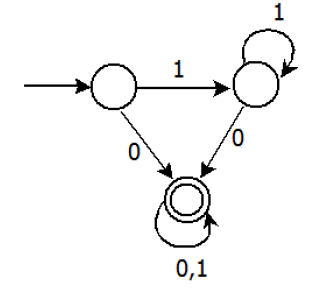Which of the following are FALSE?

 A Complement of L(A) is context-free. B L(A) = L((11*0+0)(0 + 1)*0*1*). C For the language accepted by A, A is the minimal DFA. D A accepts all strings over {0, 1} of length at least 2.
GATE CS 2013    Regular languages and finite automata
Discuss it

Question 2 Explanation:

1 is true. L(A) is regular, its complement would also be regular. A regular language is also context free. 2 is true. 3 is false, the DFA can be minimized to two states. Where the second state is final state and we reach second state after a 0. 4 is clearly false as the DFA accepts a single 0.

 Question 3

Given the language L = {ab, aa, baa}, which of the following strings are in L*?

 A abaabaaabaa B aaaabaaaa C baaaaabaaaab D baaaaabaa
Regular languages and finite automata    Regular languages and finite automata
Discuss it

Question 3 Explanation:
 Question 4

Given the language L = {ab, aa, baa}, which of the following strings are in L*?

 A abaabaaabaa B aaaabaaaa C baaaaabaaaab D baaaaabaa
Regular languages and finite automata    Regular languages and finite automata
Discuss it

Question 4 Explanation:
 Question 5

Consider the set of strings on {0,1} in which, every substring of 3 symbols has at most two zeros. For example, 001110 and 011001 are in the language, but 100010 is not. All strings of length less than 3 are also in the language. A partially completed DFA that accepts this language is shown below.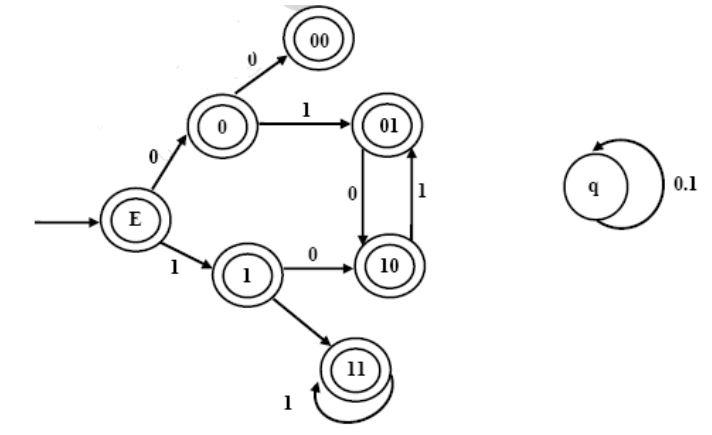The missing arcs in the DFA are

 A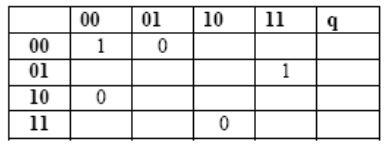B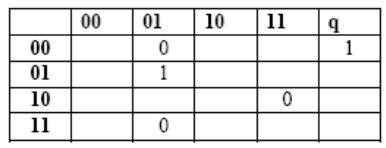C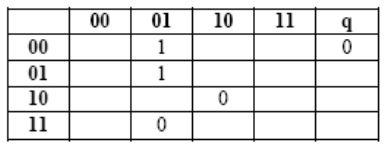D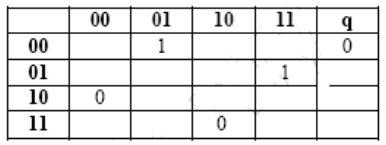GATE CS 2012    Regular languages and finite automata
Discuss it

Question 5 Explanation:
 Question 6

Definition of a language L with alphabet {a} is given as following.

             L={|ank|k>0, and n is a positive integer constant}

What is the minimum number of states needed in DFA to recognize L?

 A k+1 B n+1 C 2(n+1) D 2[Tex](k+1)[/Tex]
GATE CS 2011    Regular languages and finite automata
Discuss it

Question 6 Explanation:
 Question 7
 A A B B C C D D
GATE CS 2011    Regular languages and finite automata
Discuss it

Question 7 Explanation:
Options (B) and (C) are invalid because they both accept ‘b’ as a string which is not accepted by give DFA. (D) is invalid because it accepts "bba" which are not accepted by given DFA.
 Question 8
Let w be any string of length n is {0,1}*. Let L be the set of all substrings of w. What is the minimum number of states in a non-deterministic finite automaton that accepts L?
 A n-1 B n C n+1 D 2n-1
GATE CS 2010    Regular languages and finite automata
Discuss it

Question 8 Explanation:
We need minimum n+1 states to build NFA that accepts all substrings of a binary string. For example, following NFA accepts all substrings of “010″ and it has 4 states.Question 9
Which one of the following languages over the alphabet {0,1} is described by the regular expression: (0+1)*0(0+1)*0(0+1)* ?
 A The set of all strings containing the substring 00. B The set of all strings containing at most two 0’s. C The set of all strings containing at least two 0’s. D The set of all strings that begin and end with either 0 or 1.
GATE-CS-2009    Regular languages and finite automata
Discuss it

Question 9 Explanation:
The regular expression has two 0′s surrounded by (0+1)* which means accepted strings must have at least 2 0′s. The least possible string is ε 0 ε 0 ε = 00 The set of strings accepted is = { 00, 000, 100, 0010, 0000, 00100, 1001001,.....} We can see from the set of accepted strings that all of the have at least two zeros which is the least possible string. So option (C) is correct.
 Question 10
Which one of the following is FALSE?
 A There is unique minimal DFA for every regular language B Every NFA can be converted to an equivalent PDA. C Complement of every context-free language is recursive. D Every nondeterministic PDA can be converted to an equivalent deterministic PDA.
GATE-CS-2009    Regular languages and finite automata
Discuss it

Question 10 Explanation:
Power of Deterministic PDA is not same as the power of Non-deterministic PDA. Deterministic PDA cannot handle languages or grammars with ambiguity, but NDPDA can handle languages with ambiguity and any context-free grammar. So every non-deterministic PDA can not be converted to an equivalent deterministic PDA.
There are 155 questions to complete.
My Personal Notes arrow_drop_up